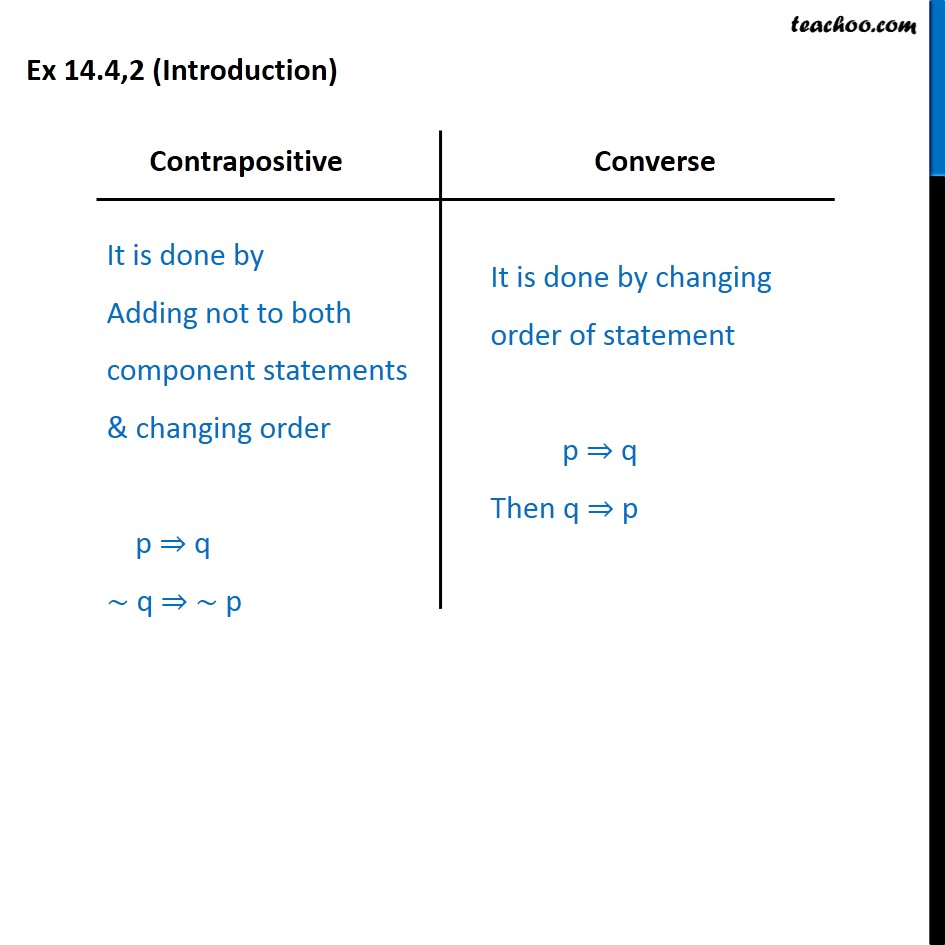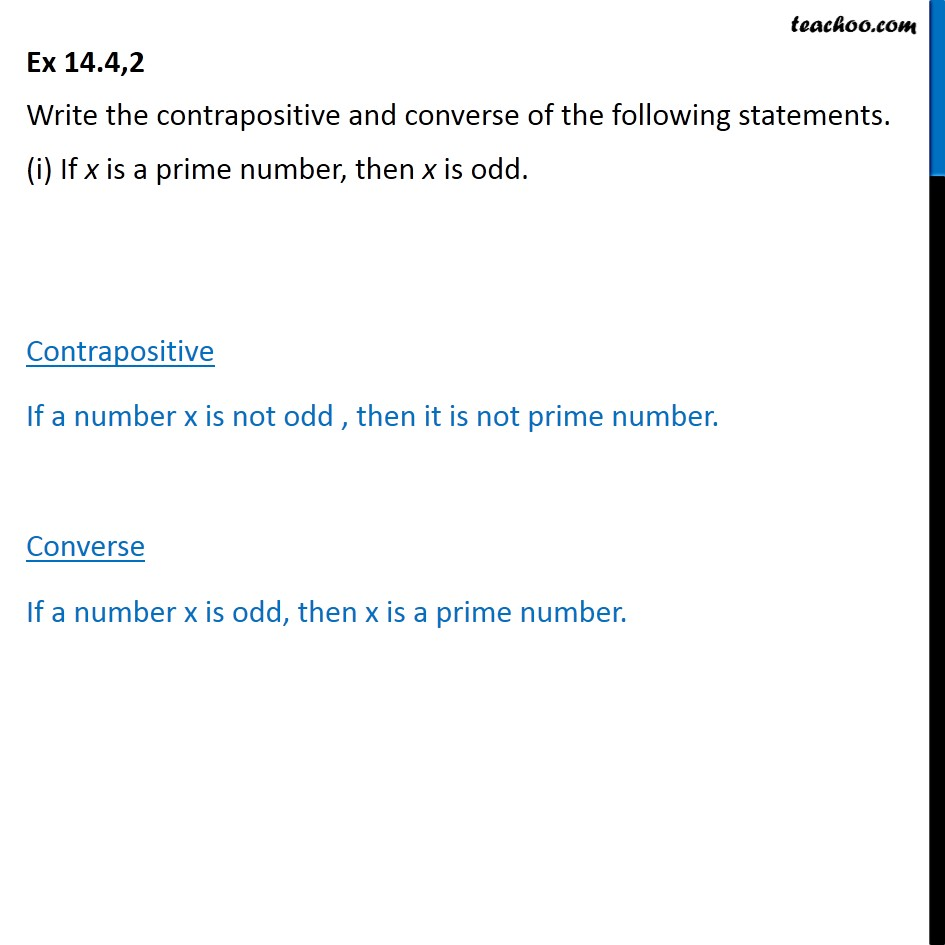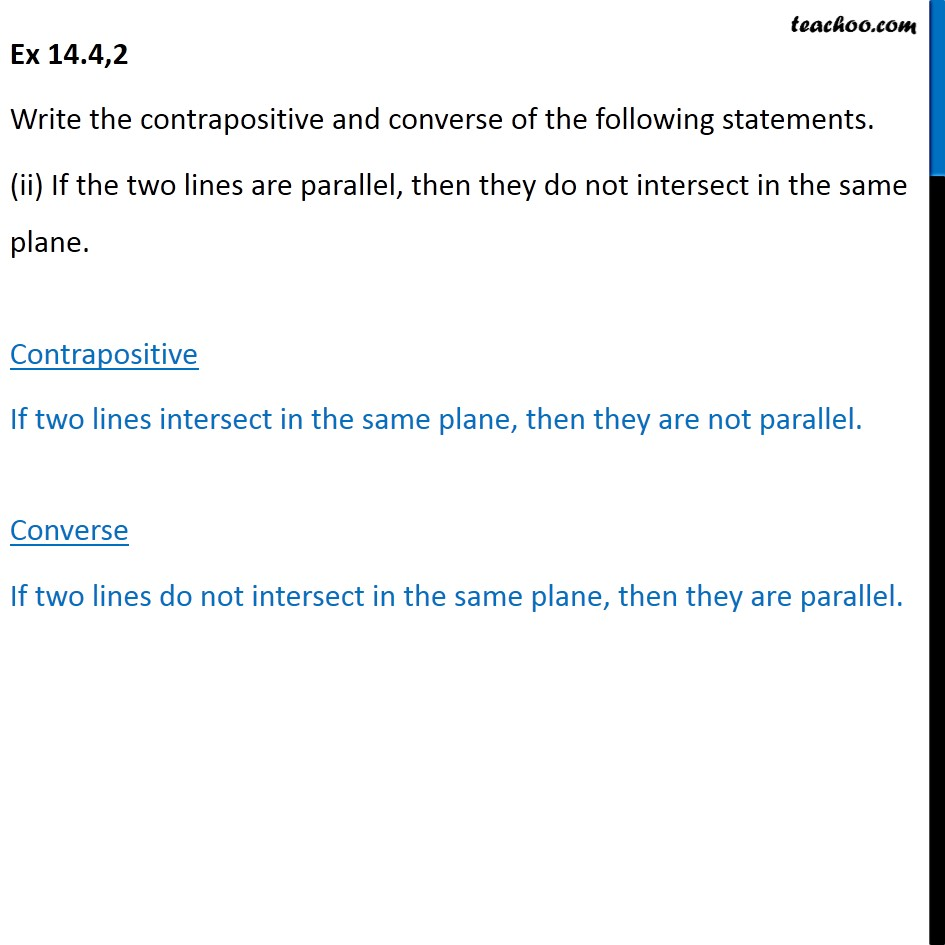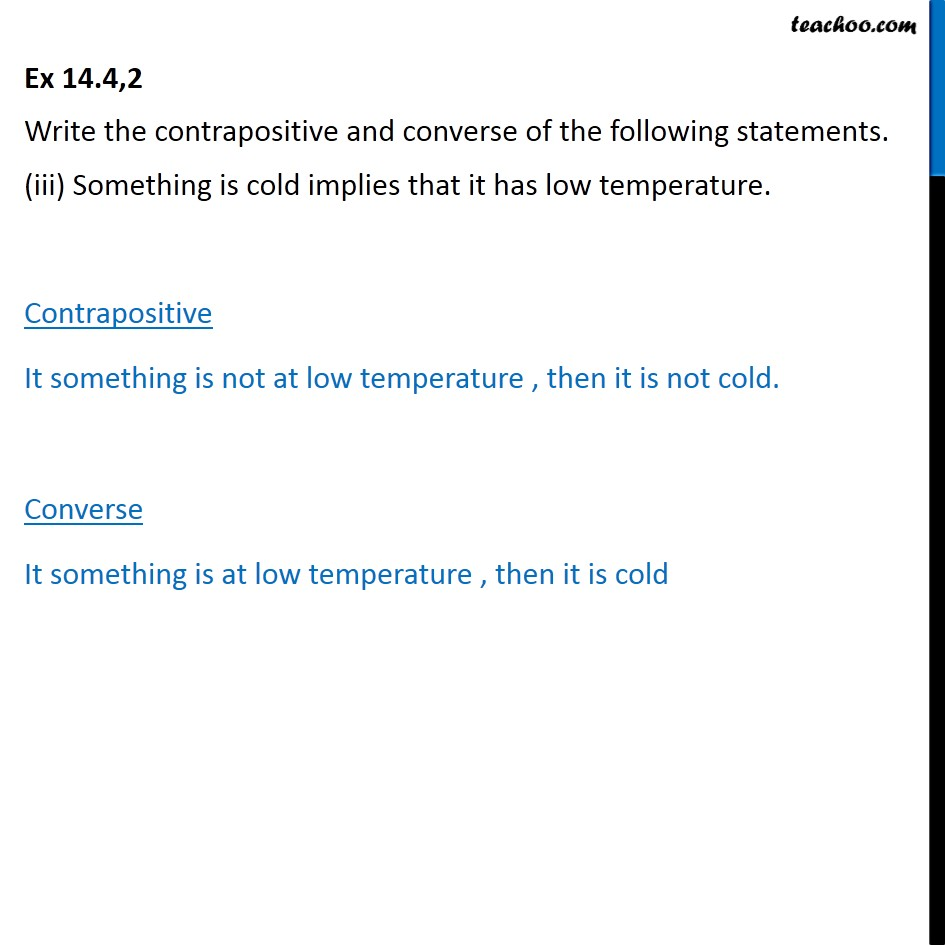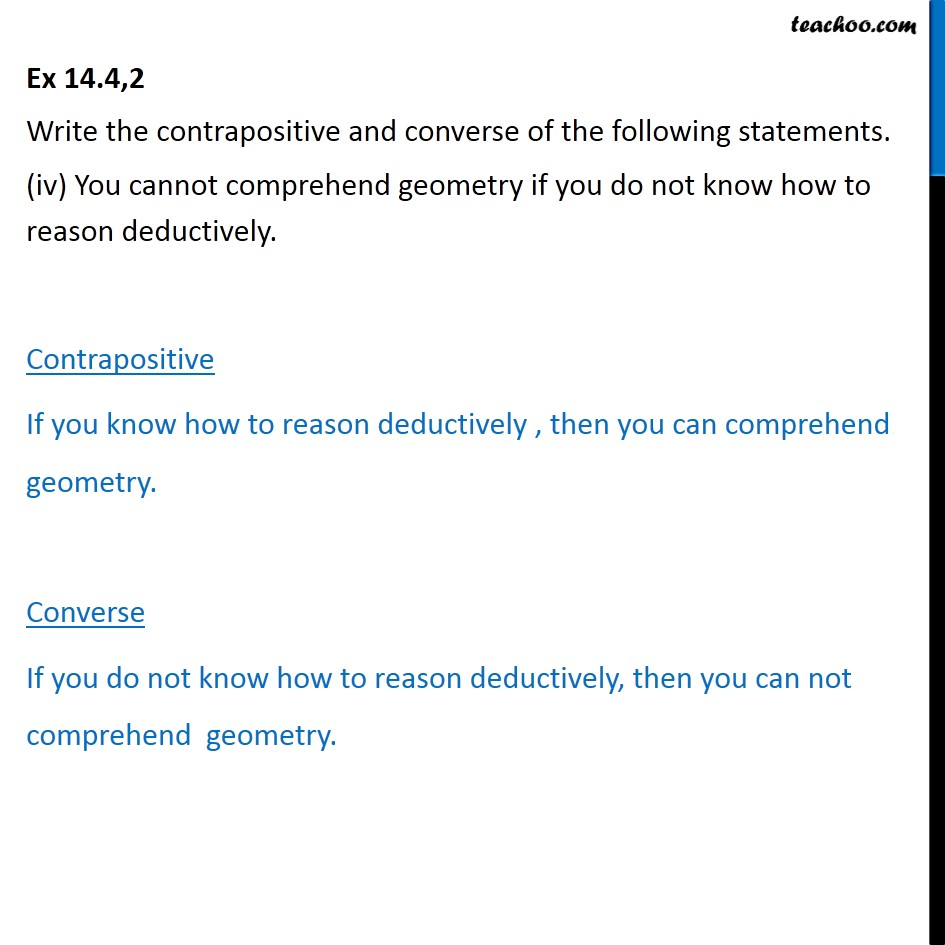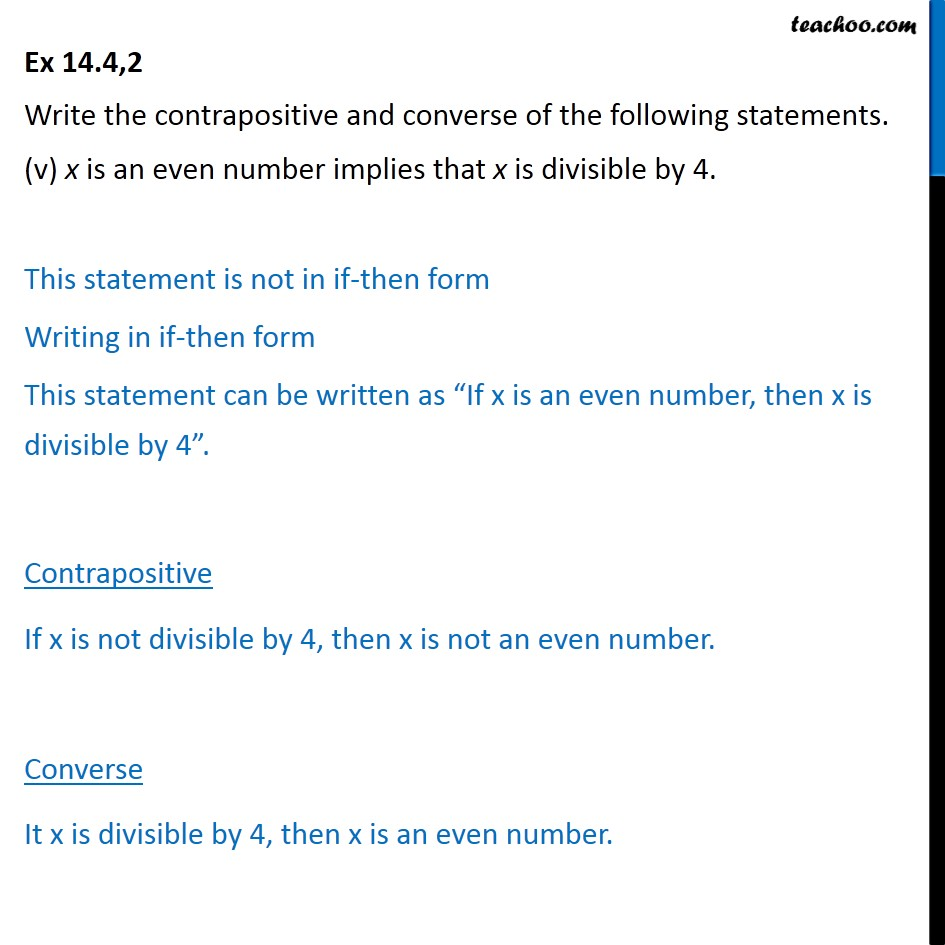Subscribe to our Youtube Channel - https://you.tube/teachoo

1. Chapter 14 Class 11 Mathematical Reasoning
2. Serial order wise
3. Ex 14.4

Transcript

Ex 14.4,2 (Introduction) Contrapositive It is done by Adding not to both component statements & changing order p ⇒ q ~ q ⇒ ~ p Converse It is done by changing order of statement p ⇒ q Then q ⇒ p Ex 14.4,2 Write the contrapositive and converse of the following statements. (i) If x is a prime number, then x is odd. Contrapositive If a number x is not odd , then it is not prime number. Converse If a number x is odd, then x is a prime number. Ex 14.4,2 Write the contrapositive and converse of the following statements. (ii) If the two lines are parallel, then they do not intersect in the same plane. Contrapositive If two lines intersect in the same plane, then they are not parallel. Converse If two lines do not intersect in the same plane, then they are parallel. Ex 14.4,2 Write the contrapositive and converse of the following statements. (iii) Something is cold implies that it has low temperature. Contrapositive It something is not at low temperature , then it is not cold. Converse It something is at low temperature , then it is cold Ex 14.4,2 Write the contrapositive and converse of the following statements. (iv) You cannot comprehend geometry if you do not know how to reason deductively. Contrapositive If you know how to reason deductively , then you can comprehend geometry. Converse If you do not know how to reason deductively, then you can not comprehend geometry. Ex 14.4,2 Write the contrapositive and converse of the following statements. (v) x is an even number implies that x is divisible by 4. This statement is not in if-then form Writing in if-then form This statement can be written as “If x is an even number, then x is divisible by 4”. Contrapositive If x is not divisible by 4, then x is not an even number. Converse It x is divisible by 4, then x is an even number.

Ex 14.4